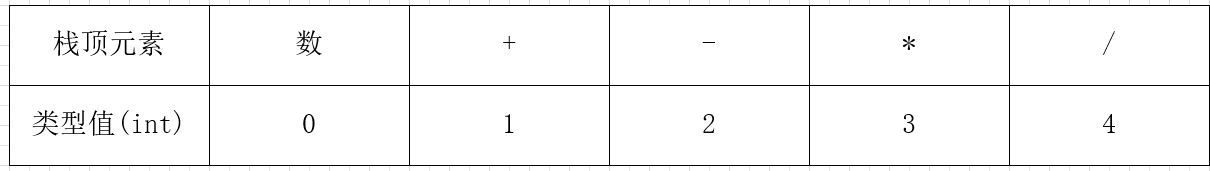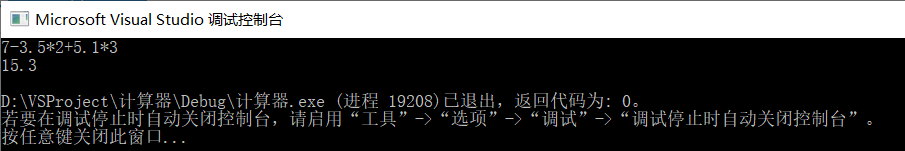• 表达式计算C++

千次阅读 2017-10-29 08:04:14
表达式计算C++可运行

原创作者：Daniel

时间：2017.10.29

地点：大连理工大学软件学院

表达式计算至少需要两个栈：1、数据栈 2、运算符栈

中缀表达式定义：

<表达式>::=<项> + <项> |<项> - <项> | <项>

项 ::= <因子> * <因子> | <因子> / <因子> | <因子>

<因子> ::= <常数> | (表达式)

<常数> ::= <数字> | <数字> <数字>

<数字> ::= 0|1|2|3|4|5|6|7|8|9

中缀表达式计算通常按照我们习惯的先乘除后加减，有括号先算括号。

逆波兰表达式定义：

<表达式>::=<项><项> + |<项>  <项> - | <项>

项 ::= <因子> <因子> *| <因子>  <因子>／ | <因子>

<因子> ::= <常数>

<常数> ::= <数字> | <数字> <数字>

<数字> ::= 0|1|2|3|4|5|6|7|8|9

在逆波兰表达式中，数字按照原来的顺序出现，运算放在参与运算的两个项或者因子后边

比如：

23 + （34 * 45）／（5 + 6 + 7）

23 34 45*  5 6 +7+／+

计算逆波兰表达式比中缀表达式要容易一些，具体过程如下：

1、扫描表达式，操作数入栈

2、遇到运算符，就在栈顶取出两个元素，用后取出的 操作 先取出的

3、将运算所得的数字进栈

4、循环123，直到表达式扫描完成，栈顶元素就是表达式的值

在计算中缀表达式时有两种方法：1、转化为后缀表达式 2、直接计算

1、中缀转化后缀方法：

1）定义操作数的栈内外优先级

2）扫描中缀表达式，遇到数字就输出

2）遇到运算符，就将预算符的优先级（栈外优先级）与栈顶元素比较

若栈外 高于 栈内 进栈

栈外 低于 栈内，依次弹出运算符输出，直到栈顶元素优先级小于或者等于栈外元素优先级

如果栈内外优先级相等，则栈顶元素弹出，不输出

2、直接计算和1 过程基本一致，需要在运算符出栈过程进行算术运算，同时需要管理两个栈。

#include<stdio.h>
#include<stdlib.h>
#include<stack>

int priorityisp(char op) {

switch (op) {
case '#':
return 0;
break;
case '(':
return 1;
break;
case '^':
return 7;
break;
case '*':
case '/':
return 5;
break;
case '+':
case '-':
return 3;
break;
case ')':
return 8;
break;
default:

printf("error!");
break;
}

return -1;
}

int priorityicp(char op) {

switch (op)
{
case '#':
return 0;
break;
case '(':
return 8;
break;
case '^':
return 6;
break;
case '*':
case '/':
return 4;
break;
case '+':
case '-':
return 2;
break;
case ')':
return 1;
break;
default:
printf("error!");
break;
}

return -1;
}

char* changeExp(char* exp) {

char* postfixExp = (char*)malloc(sizeof(char) * 20);
std::stack<char> oper;
oper.push('#');
int i = 0;
int j = -1;
while (exp[i]) {

switch (exp[i]) {
case '0':
case '1':
case '2':
case '3':
case '4':
case '5':
case '6':
case '7':
case '8':
case '9':
postfixExp[++j] = exp[i];
break;
default:
while (priorityicp(exp[i]) < priorityisp(oper.top())) {
postfixExp[++j] = oper.top();
oper.pop();
}

if (priorityicp(exp[i]) > priorityisp(oper.top()))
oper.push(exp[i]);

else  {
oper.pop();
}

break;
}
i++;
}

postfixExp[++j] = '\0';
return postfixExp;
}

int calculate(char* postfixExp) {

std::stack<char> cal;
int i = 0;

while (postfixExp[i]) {

switch (postfixExp[i]) {
case '0':
case '1':
case '2':
case '3':
case '4':
case '5':
case '6':
case '7':
case '8':
case '9':
cal.push(postfixExp[i]);
break;
default:
int num1 = cal.top() - '0';
cal.pop();

int num2 = cal.top() - '0';
cal.pop();

switch (postfixExp[i]) {

case '+':
cal.push(num2 + num1 + '0');
break;
case '-':
cal.push(num2 - num1 + '0');
break;
case '*':
cal.push(num2 * num1 + '0');
break;
case '/':
cal.push(num2 / num1 + '0');
break;
}
}
i++;
}

return cal.top() - '0';
}

int main(int argc, char** argv) {

char InfixExp;
char* PostfixExp;
int i = 0;

while ((InfixExp[i] = getchar()) != '\n')
i++;
InfixExp[i] = '\0';
PostfixExp = changeExp(InfixExp);
printf("%d", calculate(PostfixExp));
system("pause");

return 0;
}

展开全文• c++ 实现数学表达式计算 支持 常见的数学函数。如sin cos sqrt abs ... 支持 大多数运算符。 使用良好的设计模式，易于维护和拓展。
• 可实现数据结构练习——表达式自动检查和计算C++代码
• 表达式计算C++面向对象版，支持+，-，*，/，（，）运算符，支持多位小数，核心逻辑代码做了封装，可以用于CMD或者GUI下
• 计算任意表达式（整数+-*/^)的结果。原理为带优先级的符号（括号内的算式优先级加三），然后优先级从大到小遍历表达式
• #include #include #include char OP={'+','-','*','/','(',')','#'}; int In(char c){ int i; for(i=0;i;i++) if(c==OP[i]){return 1;break;} return 0; } int num(char e){ int i;... }
• 中缀转后缀表达式计算实现源码（C++、Java）
• std::string src = argc > 1 ? argv : "12+((2+73)*4)-15"; std::cout ; Expression expression; Expression::PrefixType result; int ret = expression.ToPrefix(src, result); if (ret !... }C++ 编译原理
• C++语言，利用堆栈实现波兰表达式实现后缀表达式计算
• 通过逆波兰式来计算数学中的中缀表达式，例如：3+(4-7*5)，主要是处理运算优先级问题。源码里面的注释非常详细，由于基于基本数据类型实现，实用价值较小，用作学习还是很不错的。（支持的符号（半角模式）：...
• C++后缀表达式计算

千次阅读 2019-11-19 22:32:17
C++后缀表达式计算 继续上篇文章，实现计算器简单计算的功能 上篇文章C++中缀表达式转后缀表达式已经将中缀表达式转换成后缀表达式并保存在队列q中 后缀表达式算法思想： 读取队列q顶端元素，并判断元素类型...

C++后缀表达式计算

继续上篇文章，实现计算器简单计算的功能

上篇文章C++中缀表达式转后缀表达式已经将中缀表达式转换成后缀表达式并保存在队列q中

后缀表达式算法思想：

1. 读取队列q顶端元素，并判断元素类型
(1)若是数，将数压入运算栈sum中；
(2)若是运算符，取出栈sum顶端的两个元素计算，结果压回栈sum;

2. 将队列顶端元素出栈，重复1操作，直至队列为空，此时sum中结果即为中缀表达式运算结果。

队列顶端元素对应类型值代码

#include <iostream>
#include<string>
#include <stack>
#include <queue>
using namespace std;

stack<char> s;//存放运算符的栈
queue<string> q;//输出队列（用于计算后缀表达式）
stack<double> sum;//用于计算后缀表达式
string str = "";//读取整个数字串并保存
int isp(char c)//栈内元素优先级判断
{
switch (c)
{
case '#':
return 0;
case '(':
return 1;
case '+':
case '-':
return 3;
case '*':
case '/':
case '%':
return 5;
case ')':
return 6;
}
}
int icp(char c)//栈外元素优先级判断
{
switch (c) {
case '#':
return 0;
case ')':
return 1;
case '+':
case '-':
return 2;
case '*':
case '/':
case '%':
return 4;
case '(':
return 6;
}
}
void postfix(string input)//中缀表达式转后缀表达式代码
{
s.push('#');//将#压入栈顶
input += '#';//添加#号作为结束符
for (int i = 0; i < input.length(); i++)
{
if ((input[i] >= '0' && input[i] <= '9') || input[i] == '.')
{
str += input[i];
}
else
{
if (str.length() > 0)//将数放入直接输出到队列中
{
q.push(str);
str = "";
}
while (isp(s.top()) > icp(input[i]))
{
string a;//因为不能直接在栈和队列间转换字符和字符串类型，所以先将字符转成 字符串再出栈进队列
a = s.top();
q.push(a);
s.pop();
}
//判断站外元素优先级决定元素是应该去除（=），还是进栈(<)
if (isp(s.top()) == icp(input[i]))
{
s.pop();
}
else
{
s.push(input[i]);
}
}
}
}

int wordType(string str)//队列顶元素类型
{
if (str == "+")
{
return 1;
}
else if (str == "-")
{
return 2;
}
else if (str == "*")
{
return 3;
}
else if (str == "/")
{
return 4;
}
else {
return 0;//元素数字
}
}
{
double temp1, temp2,result;
temp2 = sum.top();//先取操作数2(运算符后面的操作数)
sum.pop();
temp1 = sum.top();//再取操作数1(运算符前面的操作数)
sum.pop();
result = temp1 + temp2;
sum.push(result);
}
void subOperation()//减运算
{
double temp1, temp2, result;
temp2 = sum.top();
sum.pop();
temp1 = sum.top();
sum.pop();
result = temp1 - temp2;
sum.push(result);
}
void mulOperation()//乘运算
{
double temp1, temp2, result;
temp2 = sum.top();
sum.pop();
temp1 = sum.top();
sum.pop();
result = temp1 * temp2;
sum.push(result);
}
void divOperation()//除运算
{
double temp1, temp2, result;
temp2 = sum.top();
sum.pop();
temp1 = sum.top();
sum.pop();
result = temp1 / temp2;
sum.push(result);
}
void suffix()//计算后缀表达式，结果保留在栈sum中
{
while (q.empty() == false)
{
int objType;//队列顶元素的类型（0:数字;1:+;2:-;3:*;4:/）
objType = wordType(q.front());
switch (objType)
{
case 0:
sum.push(atof(q.front().c_str())); break;//使用c_str()方法将string转换成double型,将数字放入计算栈中
case 1:
case 2:
subOperation(); break;
case 3:
mulOperation(); break;
case 4:
divOperation(); break;
}
q.pop(); //将队列顶元素出队列
}
}

int main()
{
string input;
string och;
cin >> input;
postfix(input);
//while (q.empty() == false)//队列不为空则输出队列元素
//{
// och = q.front();
// cout << och;
// q.pop();
//}
suffix();
cout << sum.top() << endl;
}

实验结果结果正确，自行验证。

展开全文C++ 后缀表达式计算
• 后缀表达式计算，输入一个后缀表达式利用栈来计算结果后缀表达式的计算
• 开始计算 int count(Stack * top,char oper){ int v1 = pop(top); int v2 = pop(top); int result = 0; switch(oper){ case '+':result = v2 + v1;break; case '-':result = v2 - v1;break; case '*':...

1.介绍：

原理介绍https://blog.csdn.net/weixin_43386443/article/details/101615016

原理差不多,就是把结构体替换成class，和出栈进行了修改，采用出栈删除之前拿到数据并返回给调用方法。

2.源码展示：

#include <iostream>
#include <sstream>
using namespace std;
/**
@author:
@date  :
*/
class Stack{
private:
int data;				//数据
public:
Stack * next; 			//指向下一个类

void setData(int data){
this->data = data;
}
int getData(){
return this->data;
}
};

//4.出栈:删除最顶上的节点，并返回数据
int pop(Stack * top){
Stack * node;
node = top->next;
int val;
if(top->next == NULL){
return 0;
}else{
val = node->getData();
top->next = node->next;
delete(node);
return val;
}

}

//3.展示栈
void show(Stack * top){
Stack * p;
int idx = 0;
p = top->next;
do{
idx ++;
cout<<p->getData()<<endl;
p = p->next;
}while(p != NULL);
cout<<"length = "<<idx<<endl;
}

//2.入栈
void push(Stack * top,int num){
Stack * p = new Stack;
p->setData(num);
p->next = top->next;
top->next = p;
}

//5.开始计算
int count(Stack * top,char oper){
int v1 = pop(top);
int v2 = pop(top);
int result = 0;
switch(oper){
case '+':result = v2 + v1;break;
case '-':result = v2 - v1;break;
case '*':result = v2 * v1;break;
case '/':result = v2 / v1;break;
default:cout<<"Invalid character!!"<<endl;break;
}
//得到结果之后把结果入栈
push(top,result);
cout<<v2<<oper<<v1<<"="<<result<<endl;
return result;
}

//1.输入
void input(Stack * top){
string str;
int idx = 0;
int result = 0;
string num = "";
cout<<"input：";
cin>>str;
for(int i=0;i<str.size();i++){
if(str[i] == '('){		//如果被括号包裹，就视为是一个整体数，比如(10)
//记录前括下标 和后扩下表，取中间的值
idx++;
}else if(str[i] == ')'){
idx--;
i++;			//下表增加跳过）
//把值强转
int number;
stringstream ss ;
ss << num;
ss >> number;
push(top,number);
}
//如果idx不为0说明还在括号内
if(idx != 0){
//取里面的纯数字
if(str[i] >= '0' && str[i] <= '9'){
num += str[i];				//拿到括号中的值
}
}else{
if(str[i] >= '0' && str[i] <= '9'){
int val;
stringstream ss ;
ss << str[i];
ss >> val;
push(top,val);
}else if(str[i] != ')' || str[i] != '('){
result = count(top,str[i]);
}
}
}

cout<<"The calculated results are as follows:"<<result<<endl;
}

int main(int argc, char** argv) {
Stack * stack = new Stack;			//创建一个新节点
stack->next = NULL;
input(stack);
//	show(stack);
return 0;
}

3.备注：

转载请说明出处！！！

展开全文数据结构 后缀表达式 c语言 c++
• c++ 实现数学表达式计算 支持 常见的数学函数。如sin cos sqrt abs ... 支持 大多数运算符。 使用良好的设计模式，易于维护和拓展。
• 基于C++的表达式计算求值

千次阅读 2018-12-27 08:56:00
一、使用说明1.1 项目简介表达式求值是程序设计语言编译中的一个最基本的问题，就是将一个表达式转化为逆波兰表达式并求值。具体要求是以字符序列的形式从终端输入语法正确的、不含变量的整数表达式，并利用给定的...

一、使用说明

1.1 项目简介

表达式求值是程序设计语言编译中的一个最基本的问题，就是将一个表达式转化为逆波兰表达式并求值。具体要求是以字符序列的形式从终端输入语法正确的、不含变量的整数表达式，并利用给定的优先关系实现对算术四则混合表达式的求值，并演示在求值过程中运算符栈，操作数栈，输入字符和主要操作变化过程。

要把一个表达式翻译成正确求值的一个机器指令序列，或者直接对表达式求值，首先要能正确解释表达式。任何一个表达式都是由操作符，运算符和界限符组成，我们称它们为单词。一般来说，操作数既可以是常数，又可以是被说明为变量或常量的标识符；运算符可以分成算术运算符，关系运算符和逻辑运算符3类；基本界限符有左右括号和表达式结束符等。为了叙述的简洁，我们仅仅讨论简单算术表达式的求值问题，这种表达式只包括加，减，乘，除4种运算符。

人们在书写表达式时通常采用的是“中缀”表达形式，也就是将运算符放在两个操作数中间，用这种“中缀”形式表示的表达式称为中缀表达式。但是，这种表达式表示形式对计算机处理来说是不大合适的。对于表达式的表示还有另一种形式，称之为“后缀表达式”，也就是将运算符紧跟在两个操作数的后面。这种表达式比较合适计算机的处理方式，因此要用计算机来处理，计算表达式的问题，首先要将中缀表达式转化成后缀表达式，又称为逆波兰表达式。

1.2 项目功能要求

为了实现表达式求值，本项目要求首先读入表达式（包括括号）并创建对应二叉树，其次对二叉树进行前序遍历，中序遍历，后序遍历，输出对应的波兰表达式，中缀表达式和逆波兰表达式。

1.3 操作手册

运行程序后，进入欢迎界面，首先要输入表达式。

第一步，输入中缀表达式：在输入相应表达式后，会自动显示中缀、前缀、后缀表达式。参考文档和完整的文档和源码下载地址：

https://www.write-bug.com/article/1400.html

展开全文•中缀表达式
• 　编写一个程序，以字符串方式输入一个前缀表达式，然后计算它的值。输入格式为：“运算符 对象1 对象2”，其中，运算符为“+”（加法）、“-”（减法）、“*”（乘法）或“/”（除法），运算对象为不超过10的整数，...
• 字符串表达式的计算(c++版）

千次阅读 2018-10-19 19:51:20
类如: "1+8*9+(4+5)*7" 完成字符串表达式计算主要分为2个步骤; 1. 将中缀转化为后缀 按照（*/+-）的优先级；分别创建2个栈用来贮存数字和符号，从第一个元素遍历，遇到数字进入数字栈，遇到符号进入符号...j
• void deal_with_minus(char per[]){ // 处理待计算表达式里面的负号 int i,j; if(strlen(per)==1) return ; if(per=='-') { for(j=strlen(per)-1;j>=0;j--) per[j+1]=per[j]; per='0'; } ...表达式计算 中缀表达式计算
• C++实现计算器，最通俗易懂的版本, 支持括号和四则运算 ，功能完整可以直接使用使用波兰表达式进行转化。c++数学表达式解析
• 表达式计算 问题描述 　输入一个只包含加减乖除和括号的合法表达式，求表达式的值。其中除表示整除。 输入格式 　输入一行，包含一个表达式。 输出格式 　输出这个表达式的值。 样例输入 1-2+3*(4-5) 样例输出 -4 ...算法数据结构
• 按常规形式输入算术表达式（例如：输入2*(6-4)+8/4），要求能够： ⑴生成表达式的后缀表示，并输出； ⑵基于表达式的后缀表示，对该表达式求值； ⑶编写一个主程序对表达式求值函数进行测试。可直接运行
• c++字符串表达式计算

千次阅读 2020-01-07 23:13:47
先上代码 /* * @Description: Do not edit * @Author: taowentao * @Date: 2020-01-07 20:48:10 * @LastEditors : taowentao * @LastEditTime : 2020-01-07 23:12:05 ...原理是先转为逆波兰式，然后计算C++ 编译原理
• C++11中才支持lambda表达式，使用lambda，可以直接代替回调函数。不需要再去写一个函数，直接在调用的地方写代码。类似于javascript中的回调。lambda
• C++的后缀表达式计算器，运用栈，可以方便地得出浮点数运算的结果。支持的运算符有+、-、*、/、&、|、^、<（左移）、>（右移）、`（乘方）、!（整数阶乘）、\（绝对值），其中整数阶乘和绝对值是单目运算符，其它的...
• 如果了解中缀转换为后缀的程序，以及了解计算后缀表达式的程序，那么改算法就会很容易，不过是二者的结合，然后代码稍微做一点改变，基本思路不变。 1.初始化两个栈：操作数栈和运算符栈 2.若扫描到操作数，压入...
• 这是一个运行于vs2015的项目，代码也就200+行，实现的功能有基础的四则运算以及math里面cos,sin，pow函数的，有这几个函数为样本添加其他函数也很简单，代码实现简单，中缀转后缀，只用了一个栈，附加提醒，若是使用...
• C/C++ 算数表达式计算

千次阅读 2017-09-08 11:27:46
其实如果要理清编译原理之类的东西，第一步就是要搞词法分析，有了词法分析，就可以再做语法分析，我文讨论简单的词法分析的一个小例子—算数表达式，这个是我一个网友问到我的问题，虽然脑子里有个大概的思路，要么...词法分析 算数表达式...

表达式计算c++c++ 订阅